# Coding and Decoding

In this Blog we will discuss the important topic of logical reasoning I .e. coding and decoding from which a minimum of two or a maximum of five questions are asked in every Banking Examination. This test is used to judge the ability of the candidate to decode the pattern or rule which is applied at the time of coding, the information. To solve problems related to coding and decoding, candidates have to know the position of English Alphabets from Left to Right or Right to left as shown in the table below:-Coding and decoding are defined as a method of transmitting a message or signal from the sender to the receiver without a third person knowing it. On the one hand who sends the signal is called the sender and on the other hand, who receives the signal is called Receiver.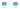Types of Coding method:-

i) Coding based on letters.

ii) Coding based on numbers.

iii) Coding based on Symbols, Digits, and Letters.

iv) Substitution Coding.

v)  Coding based on Group of word/Sentence.

vi) Coding based on mathematical operators.

i) Coding based on letters:-

Type 1:- In this type of coding the position of letter gets twisted or mixed up as shown in the example.

Example: – In a certain code ‘NUMBER’ is written as ‘MUNREB’. How is MARUTI written in that code?

Explanations:-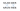In the above example, we can say that the position of the first three and last three-letter is written in reverse order. By applying the same logic.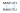Hence, MARUTI is written as RAMITU.

Type 2:- In this type of coding the original word is replaced by another word by applying certain logic or some fixed pattern.

Example: – In a certain code ‘TARGET’ is written as ‘VCTIGV’. How ‘ENGLISH’ written in the same code?

Explanation: – The Pattern given in the representation (question) shows that the original word is replaced by the second next alphabet with respect to its position.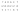Thus, by using the same logic of adding two ‘ENGLISH’ is written as ‘GPINKUJ’.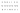ii) Coding based on numbers:-

In this type of coding, the letter of the original word is replaced by a number by applying certain logic or some fixed pattern.

Example: – In a certain code ‘AMAN’ is coded as 113114. How is ‘NEHA’ written in that code?

Explanation: –In this example, the code is based on the positions of a letter in English Alphabets, thus the logic is shown below.In a similar manner ‘NEHA’ is written as ‘14581’.Example: – In a certain code ‘NOTE’ is written as ‘4505’ and ‘BATS’  is written as ‘2109’How ‘GOAT’ written in the same code?

Explanation: – The Pattern given in the representation (question) shows that the original word is replaced by the multiplications of its alphabetical number position as shown below.Similarly, by applying the same logic the code for ‘GOAT’ is written as ‘7510’ as shown in the table below:-Example: – In a certain code. ‘BIRDS’ is coded as 52. How is ‘TIGER’ written in that code?

Explanation: –In this example, the code is based on the Addition of positions of the letter in English Alphabets, thus the logic is shown below.

The positions of letters in the word BIRDS is:Adding the position we get 52 i.e. (2+9+18+4+19), which is the code for BIRDS.

Similarly, the positions of letters in the word TIGER is:Adding the position we get 59 i.e. (20+9+7+5+18), which is the code for TIGER.

iii) Coding Based on Symbols, Digits, and Letters:

In this type of coding letters, Digits and symbols are given in the form of the table and having some relation among them. Besides these certain conditions are also given which will follow to solve the questions.

Example: – In each question given below, a group of letters followed by five combinations of letters/symbols/number i.e. 1,2,3,4 and 5.you have to find out which of the combinations represents the group of letters based on the coding system and the conditions given below, according to it mark the digits of that combination as your answer. If none of the combinations follow then mark option 5, ‘None of these’ as your answer.Conditions:-

(I)    If both the first and the last Elements (letters) in the group are vowels, both the letter is coded as #.

(II)   If both the first and the last Elements (letters) in the group are consonants, both should be coded as @.

(III)   If the first Elements (letters) is a vowel and the last Elements (letters) in the group are consonants, both should be coded as the code for the vowel.

Example:BUNRJk

(1) 502917

(2) @[email protected]

(3) 24301#

(4) #1281\$

(5) None of these

Explanation:- We know that in English alphabets a, e, i, o, u. letters are vowels and remaining letters are consonants. In the given word first and the last letter is consonant so both are replaced by @ (a condition I), and remaining letters take the same code. Therefore the answer is an option (2).

Example: – UBRKME

(1) #2681#

(2) 570812

(3) 709824

(4) 078926

(5) None of these

Explanation: – We know that in English alphabets a, e, i, o, u. letters are vowels and remaining letters are consonants.In the given word first and the last letter is Vowels so both are replaced by # (condition II), and remaining letters take the same code. Therefore the answer is an option (1).

Example: – UJIEKM

(1) 026#81

(2) 081572

(3) 982704

(4) 304783

(5) None of these

Explanation:-We know that in English alphabets a, e, i, o, u. letters are vowels and remaining letters are consonants. In the given word the first letter is Vowels and the last letter is consonants so both are replaced by the code of vowel i.e. 3 (condition III), and remaining letters take the same code. Therefore the answer is an option (4).

Example:RKBQMI

(1) 502917

(2) 682514

(3) @4321#

(4) #[email protected]

(5) None of these.

Explanation: – We know that in English alphabets a, e, i, o, u. letters are vowels and remaining letters are consonants. In the given word first letter is consonant and the last letter is vowel so it does ‘not follow any of the above conditions, hence letters take the same code as given in the table. Therefore the answer is an option (2).

1. iv) Substitution Coding:-

In Substitution Coding questions, the specific word is substituted as code names and then the question is asked for a specific word that is to be answered in the code language.

Example: -If Chess is called badminton, badminton is called hockey, hockey is called cricket and cricket is called basketball, then in which game a person use bat for playing?

3. Chess
4. None of these
5. Cricket

Explanation: – As we knew a person uses the bat for playing cricket. Thus in the given code language cricket is called basketball. Therefore, the correct answer is option 2. i.e. Basketball.

1. v) Coding Based on Group of word/Sentence:-

Coding-decoding questions are also tested for a group of words, in which three or more sentences are given in the coded language and the code for a specific words is asked, to find the code for the specific word, pickup two sentences compare them and find a common words and the common code word between the two-sentence will represent that word. Similarly, we can determine a specific code word for each word of sentences.

Example: To solve the question below, study the following information carefully and answer the questions.

In a certain code language:-

1. ‘Chalks are board’  is written as ‘se de ro’
2. ‘Board is pencil’    is written as ‘vi ne de’
3. ‘Pencil are book’    is written as ‘se ra vi’

Explanation:-

Step I– find the common words in the given sentences and their code by comparing (I) and (II).

Word                                                  code

Board                                                 de

Step II– find the common words in the given sentences and their code by comparing (II) and (III).

Word                                                  code

Pencil                                                 vi

Step III– find the common words in the given sentences and their code by comparing (III) and (I).

Word                                                  code

are                                                       se

Step IV– find the code for the words which is uncommon by comparing with its code shown as follows:-

Word                                                  code

Chalks                                                 ro

is                                                         ne

Book                                                   ra

Question 1:- What is the code for pencil?

a) ro b) ri c )ne d) vi e) di

Answer: As a pencil will be coded as ‘vi’, the correct answer is option‘d’.

Question 2:- How will ‘chalks are pencil’ be written in this code?

a) ro  di ne b) ri vi ne c ) ro se vi d) ne vi ro e) none of these

Answer:- As ‘chalk are pencil’ will be coded as  ‘ro se vi’ the correct answer is option ‘c’.

Question 3: what does ‘ne’ represent in this code?

a) Chalks   b) is c ) Book d) Board e) none of these

Answer: – As ‘ne’ will be coded as ‘is’ the correct answer is option ‘b’.

Question 4: what does ‘Board’ stand for in this code?

a) None of this b) de c ) ne d) vi e) di

Answer:- As ‘Board’ will be coded as ‘de’ the correct answer is option ‘b’

Question 5: which of the following is written as ‘de ne vi’?

a) Chalks   Board pencil  b) None of these c) Board is pencil   d) Book Board pencil e)

Answer: – As ‘de ne vi’ will be coded as ‘Board is pencil’ the correct answer is option ‘c’.

vi) Coding based on mathematical operators:-

In this type of coding mathematical operators i.e. {(plus (+), multiplication (×), Division (÷), subtraction (-)}.be coded as Alphabets.

Example: If A means plus (+), B means subtraction (-), C means multiplication (×), D means Division (÷) then find the value of given expression 20B10C30D20A15=?

Explanation:-

20B10C30D20A15=?

(By using Bodmas Rule https://www.sevenmentor.com/simplification-and- approximation/)

= 20-10×30÷20+15

=20 -10×30÷20+15

=20-15+15

= 20